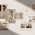## Thursday, 13 February 2020

### Custom Flight Controller Part 2.3.2: Sensor Fusion --- (Optional) Orientation Visualization Using Matlab

This post records down the steps that I did to achieve the final matlab simulation as well as discusses limitations of this method and suggests future improvements.

From previous steps I was able to obtain the data from IMU sensors and implemented a quaternion Kalman Filter for orientation calculation in matlab. The final step is therefore to use matlab to perform orientation visualization so as to prove that this the proposed algorithm can successfully keep track of the device's orientation.

Matlab provides existing functions for serial communication. The official tutorial could be found here. Again,my script could be found in my Github. In this post, I will try to explain the essential parts of my code.

This screenshot below is from serial_func.m and it demonstrates how to initialize a serial communication in matlab and configure its relevant parameters.Note that I have added in a callback function for serial communication. The last two lines of the code shown above essentially tells the program to execute the function named callback whenever a new line is detected.
Remember that the data output by arduino is of the following format as described in my previous post.

Therefore, I implemented the following functions in callback.m to extract the data.

The elaspedTime is measured in microseconds, therefore it has to be translated to seconds for calculating the rotation angles. The reason to add another 10 millisecond on top of the measurement is that I later found out the matlab simulation is not fast enough. My matlab cannot complete executing the filter and updating the simulation graph before the next data arrives. Therefore, I have to arbitrary decrease the data publication frequency of arduino by adding delay(10) at the end of the loop() in Arduino. Therefore, the elapsedTime, aka the time for which a single gyro data is valid is arbitrarily lengthened by 10 millisecond.

Note that it is not desirable as it exacerbates the problem described in my previous post, "getting data from MPU9250", that this method could only track orientation correctly if the IMU is rotated slowly. The way to fix it is to use another method of simualation, most likely Processing. For that, I have to migrate the filter from matlab to microcontroller. Since arduino does not have a floating point unit, I plan to purchase STM32 microcontroller. I will publish further posts in the future.
Nonetheless, as long as the IMU is not rotated fast, this method is sufficient to demonstrate that the quaternion Kalman Filter works.

Another additional function that I have added in to my filter was to check whether the IMU was experiencing external linear acceleration. External linear acceleration on top of the gravity will contaminate the accelerometer measurement and therefore the orientation updated. There are several methods to overcome this problem. I chose the easiest and most straight-forward, threshold switching. If the magnitude of accelerometer measurement exceeds the gravity by a certain threshold, it is interpreted as that external linear acceleration is present. Under this situation, accelerometer measurements will not be used to update the prediction given by gyroscope. This is achieved by setting the Kalman Gain of the accelerometer measurement to be zero matrix as shown below:

After getting the data, simply execute the filter by passing all relevant measurement into quaternion kalman filter that has been developed previously along with the extAcc flag.
The result given by the quaternion kalman filter would be the updated state, that is a quaternion representing the current orientation. Executing quat2eul() function in matlab will generate a rotation matrix based on the given quaternion. My method of simulating the orientation in matlab is to first draw a horizontal rectangle in matlab figure, then rotate the rectangle by premultiplying its coordinates with the rotation matrix generated. The result rectangle shown in the graph therefore should represent the final orientation of the IMU device, again, as long as the IMU is not rotated too fast.

Note that at about second, error occurred because I rotated the device too fast. Nonetheless, the simulation shows that the implemented algorithm can track the orientation relatively accurately.

However, the results demonstrated is far from being satisfactory. The current performance of the filter can not be implemented on quadcopter. Therefore, I will migrate the whole algorithm to STM32 Microcontroller. The Quoternion Kalman Filter will be re-written in C++ and run by the microcontroller directly instead of Matlab. Processing will be used to do do the same orientation visualization. Remember the most prominent problem faced here is that gyroscope data is assumed to be valid far longer than its real value. With the microcontroller having real-time operation system, this problem could be solved. I expect a better result then.

Nonetheless, I think it is safe to draw the conclusion that there is no problem in the implementation of Quaternion Kalman Filter. Since I am focusing on the software part of the custom flight controller first, I will not proceed to buy the STM32 microcontroller and write the code there immediately. Instead, I plan to focus on the flight control algorithm in this upcoming December.

I hope that I can post some fruitful results soon.

1.Nice work so far! Keep it up and good luck!

1.Yes, sensei

2."hello nice work, but can i ask some question aboute your program, why i get this eror message when i try to run your program in matlab 2016....please email the answer to email cnurizati@gmail.com
thak u"

Index exceeds matrix dimensions.

Error in callback (line 13)
mag = (IMUdata(7:9))';

Error in instrcb (line 42)
feval(val{1}, obj, eventStruct, val{2:end});

Error in Serial_func (line 48)
pause;

Warning: The BytesAvailableFcn is being disabled. To enable the
callback property
either connect to the hardware with FOPEN or set the
BytesAvailableFcn property.

> In Serial_func (line 48)